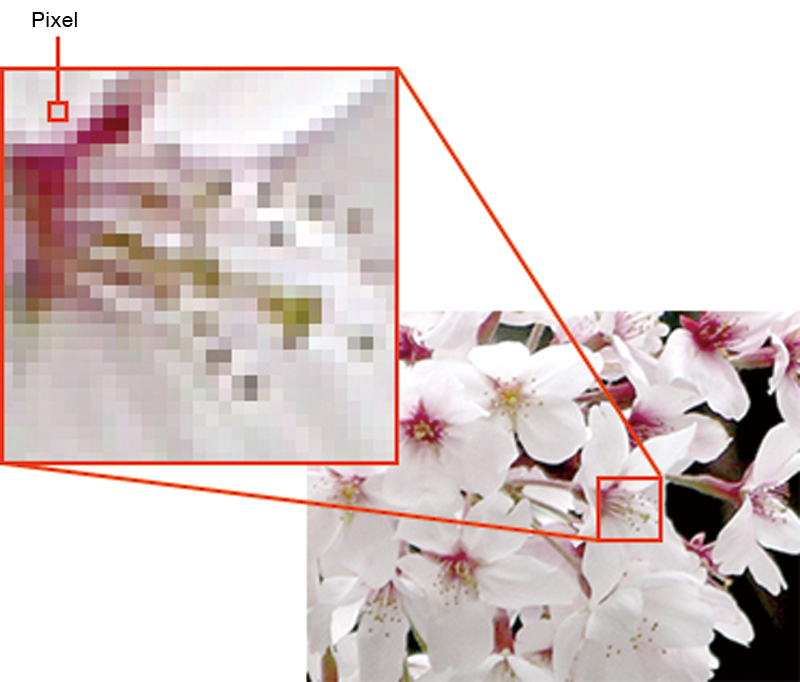# Pixel

Pixel

## CATEGORIES

A pixel is the smallest element in the composition of bitmap data. It is an abbreviation of the word, picture element, and the word for it in Japanese is gaso.

Digitized bitmap data images read by scanner or from digital camera photographs are typically comprised of a matrix of small squares and those squares are called pixels. Therefore, the actual size of the bitmap data is expressed solely by the number of pixels in that matrix (e.g. 1,200 x 1,600 pixels).

The size of one pixel is determined by the resolution. The resolution units are expressed by the number of pixels in an inch (about 25.4mm); either ppi (pixels per inch) or dpi (dots per inch). The higher the resolution, the smaller the size of the pixel and the lower the resolution, the larger the size of the pixel.

The quantity of color information in the pixel is known as color depth (or bit depth). Color depth is the number of colors (gradation) that can be expressed by the pixel. If the color depth is one bit, the pixel can express 2 colors and if the color depth is n bits, it can express 2n colors (e.g. 8-bit color depth = 28 = 256 colors. 24-bit color depth = 224 = approximately 16.77 million colors.)

When digitizing analog images, they must pass through two processes, sampling and quantization. Sampling precision settings determine the resolution and quantization precision settings determine the color depth.

•